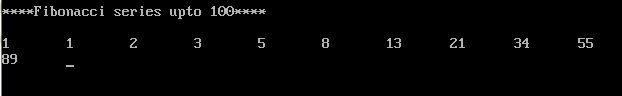UncleCoder.com

UncleCoder.com

Free programming examples and instructions

# C program to print Fibonacci series up to 100

## C Program to for how to find Fibonacci series up to 100

by Krishna

Posted on 27 Jul 2018 Category: C Views: 15319

C program to print Fibonacci series up to 100

This program shows how to get the Fibonacci series up to 100. The program for the same is given below

``````#include <stdio.h>
#include <conio.h>
void main()
{
int a=1,b=1,c=0,i;
printf(“****Fibonacci series upto 100****\n”);
printf(“%d\t%d\t”,a,b);
for(i=0;i<=10;i++)
{
c=a+b;
if(c<=100)
{
printf(“%d\t”,c);
}
a=b;
b=c;
}
getch();
}

``````

OUTPUT